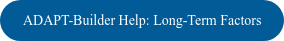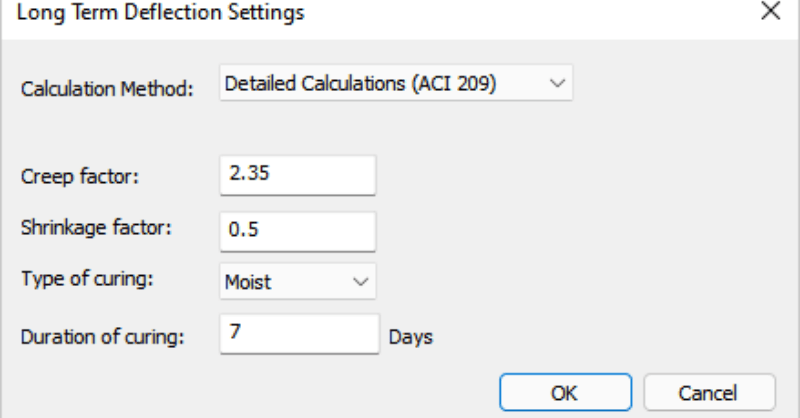With the release of ADAPT-Builder v21, users now has the option to have the program automatically calculate long-term load factors using two different methodologies. Calculation of Long-Term Load Factors is available using all design codes but is implemented based on ACI 209. When calculating these factors, either the Detailed Calculations Method (ACI 209) or the ACI Multiplier Method can be selected. When using the Detailed Calculations method, the program considers the following factors when determining the factors to apply to the long-term combinations created by the user.

• Creep

• Shrinkage

• Type & Duration of Curing

• Deflection Observation Day

When using the ACI Multiplier method, the program interpolates the long-term multiplier entered by the user based on the day deflection is observed.

To access the feature, a new Long-Term Deflection Settings option is available under the criteria menu. Selecting this option opens the Long-Term Settings window. Users are then presented with the option to select from the two methodologies mentioned above.

#### Detailed Calculation (ACI 209)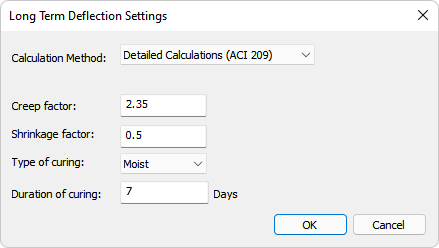The use of the Detailed Calculations (ACI 209) is typical when sustained loads are applied in stages. The program uses the user inputs from the Long-Term Deflection Settings window to calculate the creep and shrinkage coefficient, C. The coefficients are calculated as follows: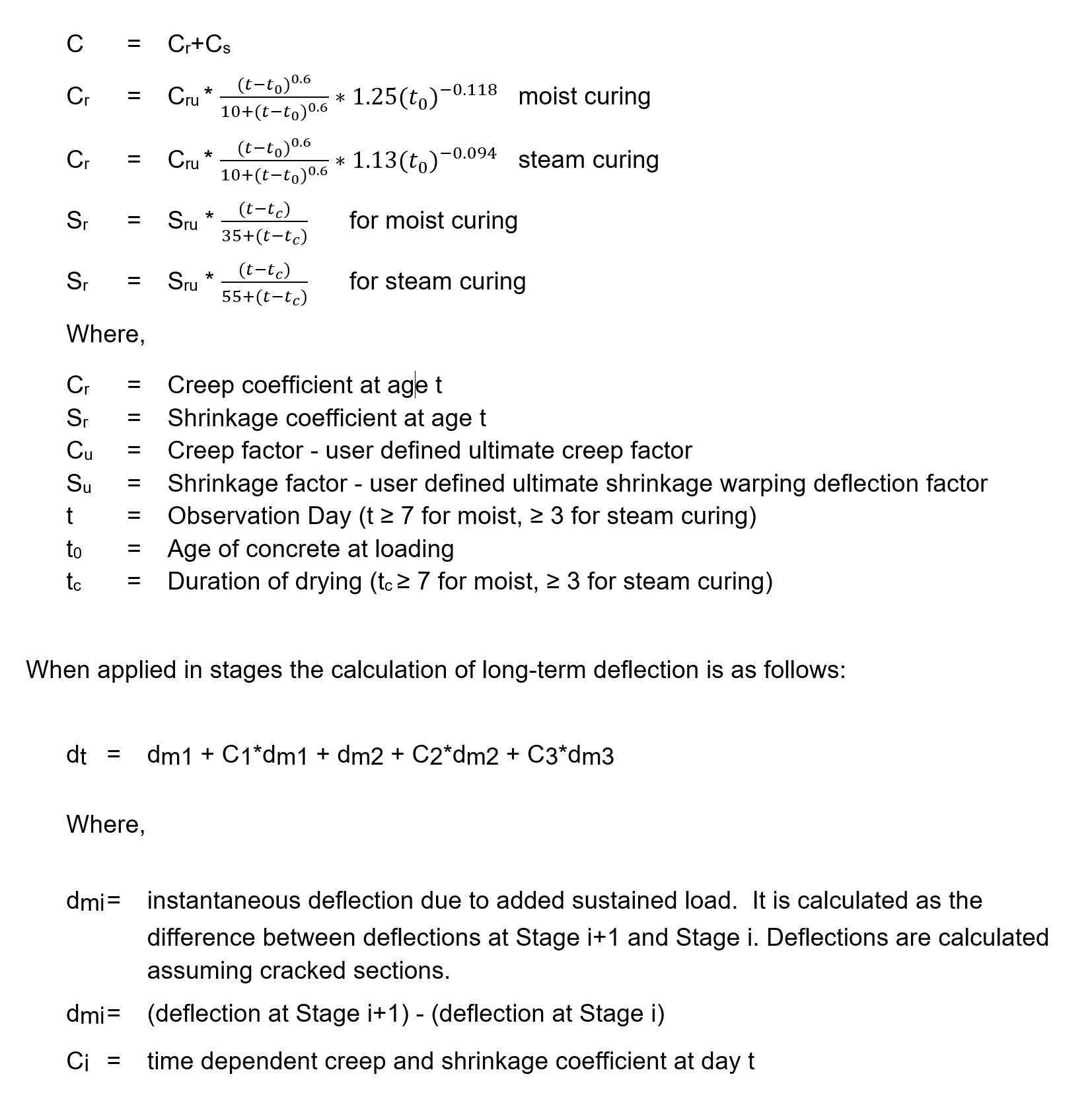#### ACI Multiplier Method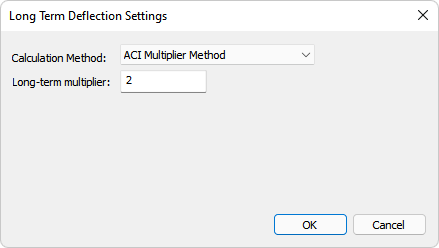The use of the ACI Multiplier Method is typical when sustained loads are applied at the same time. The program interpolates the load factors based on the Long-Term Multiplier and the date of observation for the deflection. Using the ACI Multiplier method the long-term deflection is calculated as: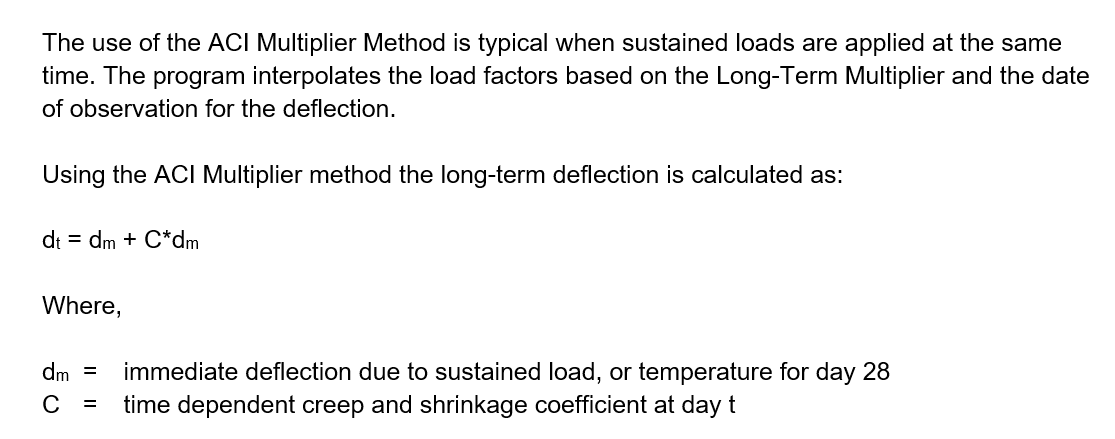#### Auto Calculate Long-Term Load Factors

To have the program automatically calculate load factors, open the Long-Term Deflection Settings window by clicking on the Long-Term Settings icon on the Criteria ribbon Design Criteria panel.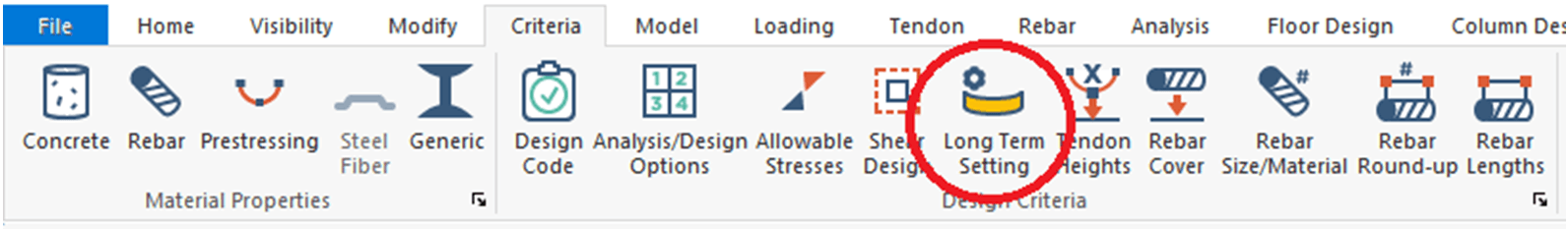#### Example

The user can then choose the Calculation Method within the Long-Term Deflection Settings and complete the user inputs required for that method. In this example we will use the ACI Multiplier method assuming that sustained loads are applied at the same time with a long-term multiplier of 2 as shown in the following figure.Once the Long-Term Deflection Settings are selected, the user can then setup the sustained load combination and the long-term load combination to be used to evaluate deflection within the model. An example of typical load combinations created for this method are shown below. Note that the "Age at Loading" and the "Observation Day" in the load combination inputs as well.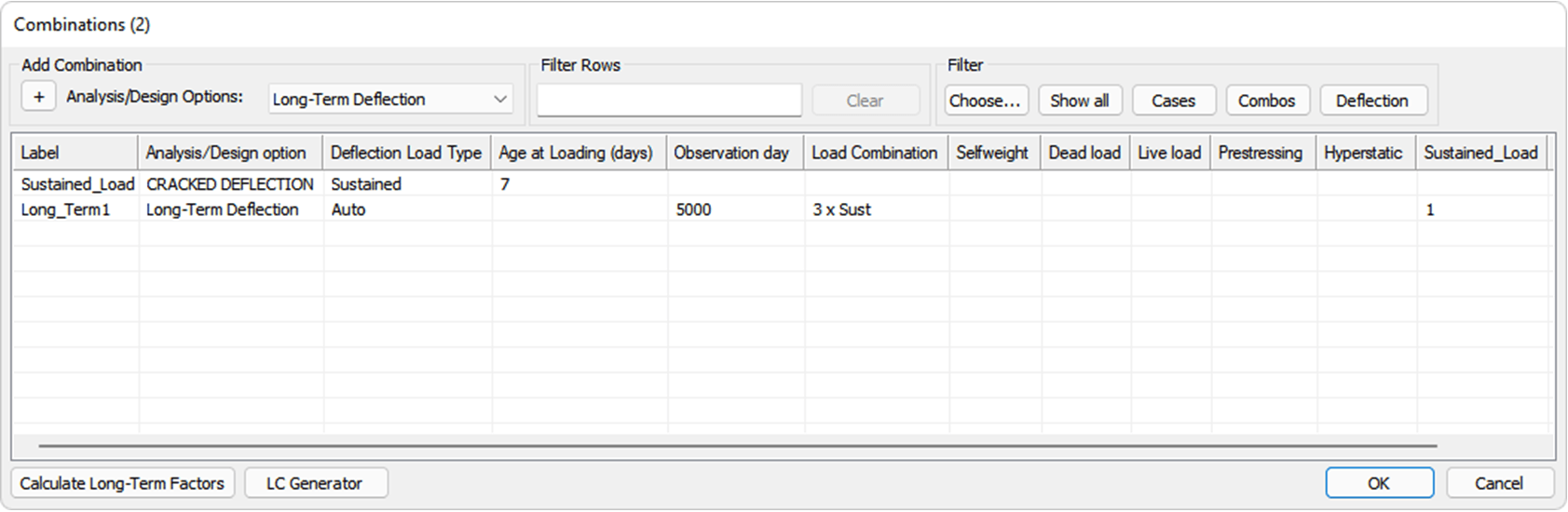Notice that for the Sustained_Load load combination we have entered a dummy factor of 1.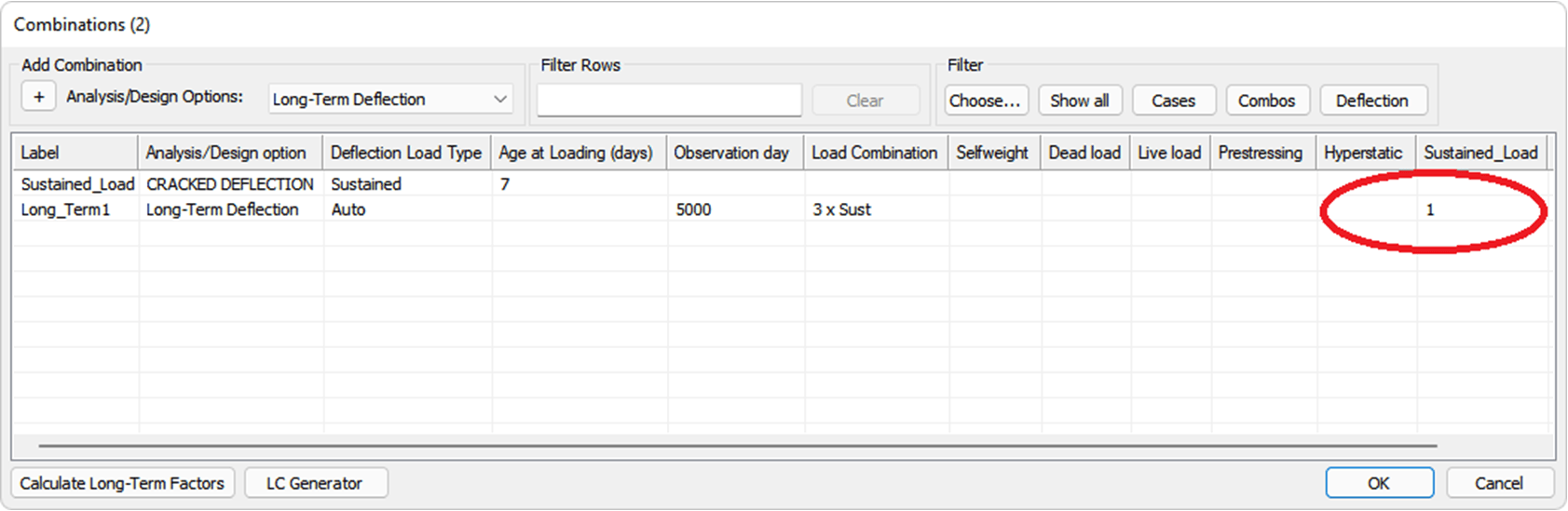We can now click on the Calculate Long-Term Factors button in the lower left corner.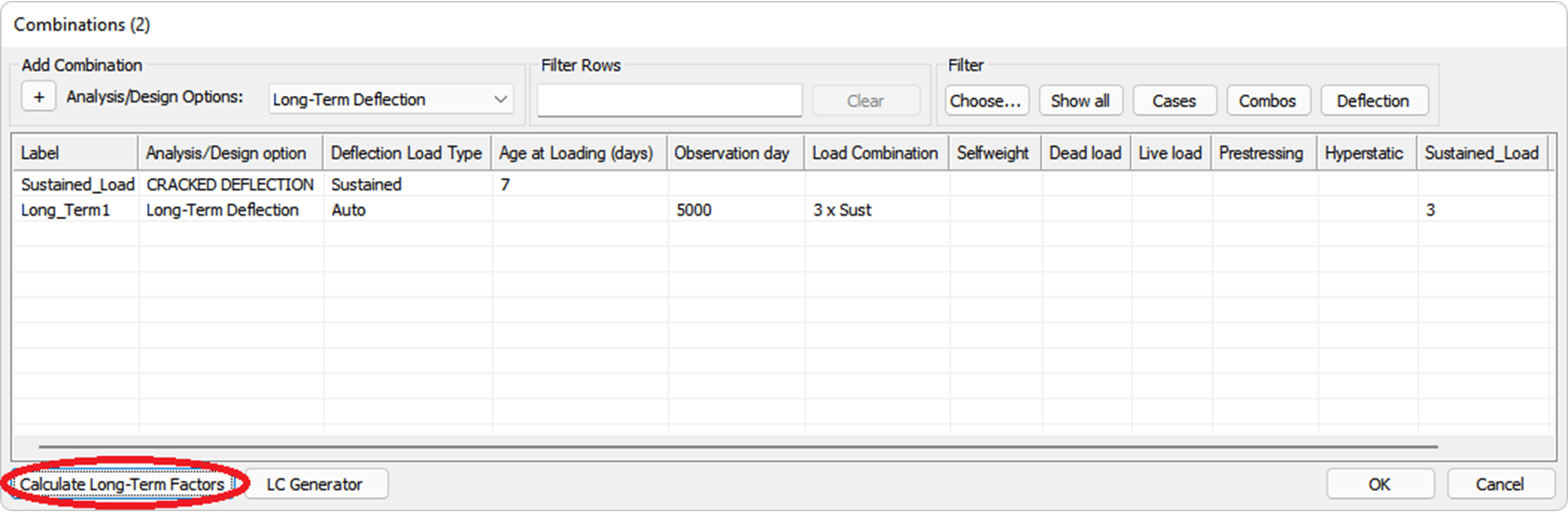The Sustained_Load load factor will automatically update based on the Calculation Method selected by the user in the Long-Term Deflection Settings dialogue.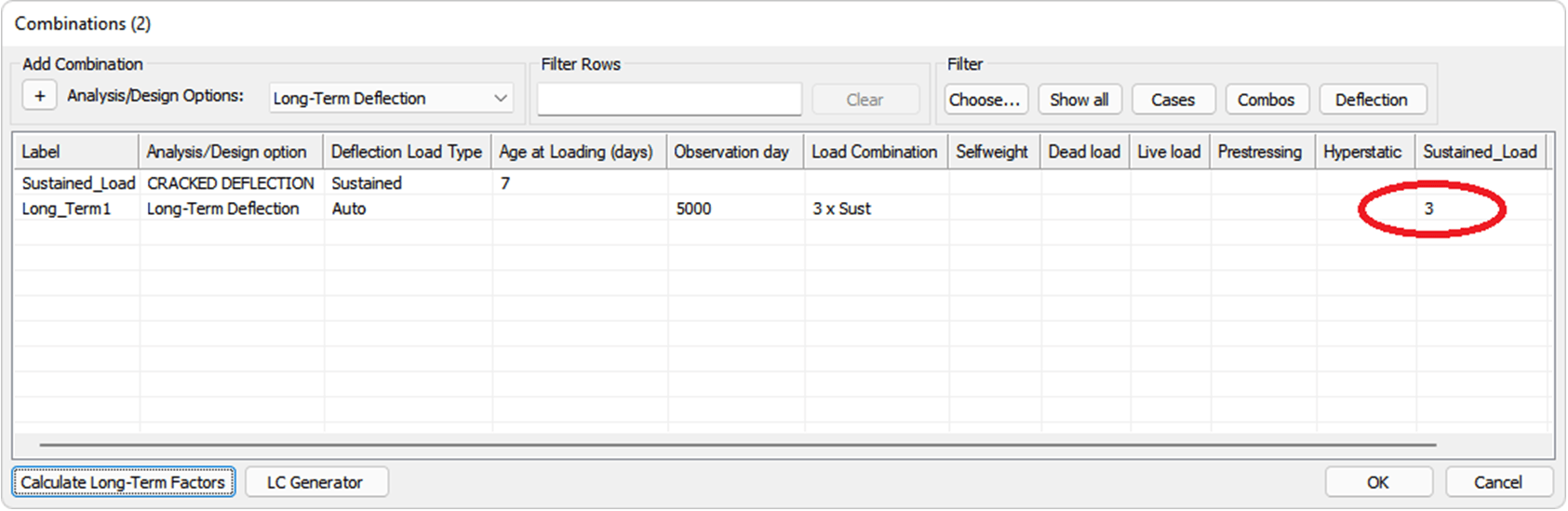The same procedure would be followed using the Detailed Calculation Method resulting in an even more accurate prediction of deflections for the day deflections are observed. To learn more about the automatic calculation of long-term deflection factors, review the ADAPT-Builder Help file and check out the video below.